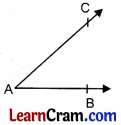# DAV Class 4 Maths Chapter 10 Worksheet 1 Solutions

The DAV Maths Book Class 4 Solutions and DAV Class 4 Maths Chapter 10 Worksheet 1 Solutions of Angles offer comprehensive answers to textbook questions.

## DAV Class 4 Maths Ch 10 WS 1 Solutions

Question 1.
Tick (✓) the figures where an angle is formed.(b), (c) and (g)

Question 2.
Draw any four angles in your notebook.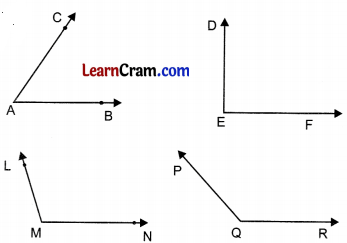### DAV Class 4 Maths Chapter 6 Worksheet 1 Notes

Point:
A point is an exact location.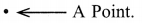Line segment:
Let A and B are two points.The straight path from A to B is called the line segment AB. It is denoted by $$\overline{A B}$$.

Line:
A line is an endless straight path that extends in both directions. A line has no end points. It is denoted by $$\overline{A B}$$.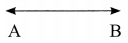Ray:
A ray is a straight path having one end point and extending endlessly in one direction only. It is denoted by $$\overline{A B}$$.Acute Angle:
The angle whose measure is less than 90° is called an acute angle.

Obtuse Angle:
The angle whose measure is more than 90° is called an obtuse angle

Right Angle:
The angle whose measure is equal to 90° is called as right angle.

Straight Angle:
The angle of 180° is called as straight angle.

Question 1.
Identify the following as ray, line or line segment.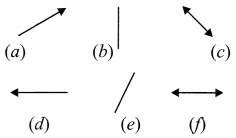(a) Ray
(b) Line segment
(c) Line
(d) Ray
(e) Line segment
(f) Line.

Question 2.
How many line segments are there in each?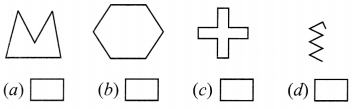(a) 5
(b) 6
(c) 12
(d) 7Question 3.
Match the following:

 A line segment has one end point → represents a no end points A ray has two end points A line segment is a part ray A line has measured A line segment can be of a line

 A line segment has two end points. → represents a ray A ray has one end point A line segment is a part of a line A line has no end points A line segment can be measured.

Question 4.
Construct the following line segments.
(a) XY = 3 cm(b) CD = 4 cm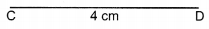(c) AB = 12 cm